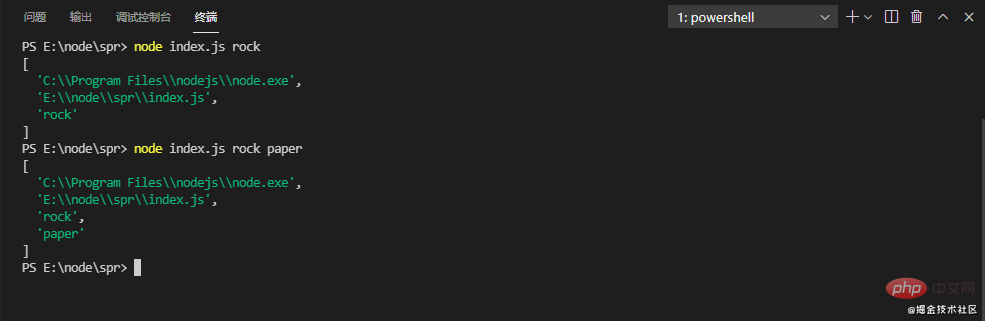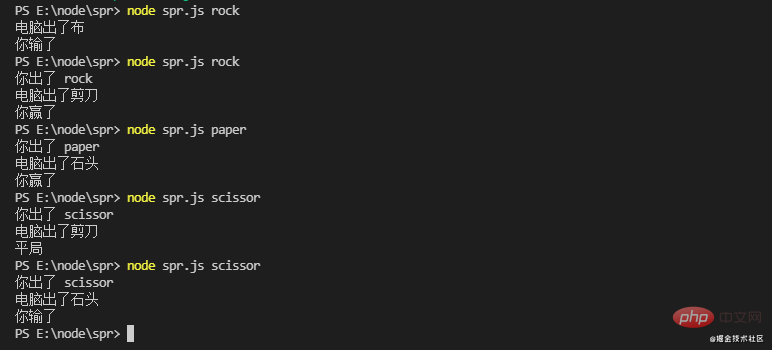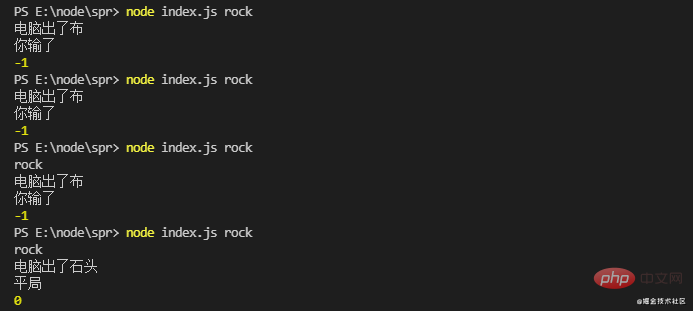用户名 Email 自动登录 找回密码 密码 立即注册

# 使用Nodejs如何实现一个简单的石头剪刀布小游戏### 需求分析

`console.log(process.argv)`### 初始版本

```// spr.js
// 石头剪刀布小游戏
// 命令行命令：node spr.js rock
// argv 可以获取到命令行 node 后面输入的行为
// let playerAction = process.argv[process.argv.length - 1]; // 最后面的输入
let playerAction = process.argv; // 也可以通过下标直接获取
console.log('你出了', playerAction)
if (playerAction != 'rock' && playerAction != 'paper' && playerAction != 'scissor') {
console.log('请输入rock或paper或scissor')
} else {
// 电脑通过随机数生成石头剪刀布
let computerAction;
let random = Math.random() * 3;
if (random < 1) {
console.log('电脑出了石头')
computerAction = 'rock'
} else if (random > 2) {
console.log('电脑出了剪刀')
computerAction = 'scissor'
} else {
console.log('电脑出了布')
computerAction = 'paper'
}

// 比较分出输赢
if (computerAction === playerAction) {
console.log('平局')
} else if (
(computerAction == 'rock' && playerAction == 'scissor') ||
(computerAction == 'scissor' && playerAction == 'paper') ||
(computerAction == 'paper' && playerAction == 'rock')
) {
console.log('你输了')
} else {
console.log('你赢了')
}
}```

```node spr.js rock
node spr.js scissor
node spr.js paper```### 模块封装

```// game.js
module.exports = function (playerAction) {
if (['rock', 'scissor', 'paper'].indexOf(playerAction) == -1) {
throw new Error('请输入rock或paper或scissor');
}
// 电脑通过随机数生成石头剪刀布
var computerAction;
var random = Math.random() * 3
if (random < 1) {
computerAction = 'rock'
console.log('电脑出了石头')
} else if (random > 2) {
computerAction = 'scissor'
console.log('电脑出了剪刀')
} else {
computerAction = 'paper'
console.log('电脑出了布')
}

// 比较分出输赢
if (computerAction === playerAction) {
console.log('平局')
return 0;
} else if (
(computerAction == 'rock' && playerAction == 'scissor') ||
(computerAction == 'scissor' && playerAction == 'paper') ||
(computerAction == 'paper' && playerAction == 'rock')
) {
console.log('你输了')
return -1;
} else {
console.log('你赢了')
return 1;
}
}```

```// argv 可以获取到命令行 node 后面输入的行为
var playerAction = process.argv[process.argv.length - 1];
console.log(playerAction);

// 通过 require 引入石头剪刀布游戏模块
const game = require('./game.js')
const result = game(playerAction);
console.log(result)``````// 获取进程的标准输入
process.stdin.on('data', (buffer) => {
// 回调的是 buffer，需要处理成 string
const action = buffer.toString().trim();
console.log(action)
})``````const game = require('./game.js')
var winCount = 0;
// 获取进程的标准输入
process.stdin.on('data', (buffer) => {
// 回调的是 buffer，需要处理成 string
const action = buffer.toString().trim();
const result = game(action);
if (result == 1) {
winCount++
if (winCount == 3) {
console.log('我不玩儿了！哼！');
process.exit();
}
}
})```推荐文章
• 热门
• 最新